# Using Forest Plots to Report Regression Estimates: A Useful Data Visualization TechniqueOctober 17, 2023

## Using Forest Plots to Report Regression Estimates: A Useful Data Visualization Technique

Regression models help us understand relationships between two or more variables. In many cases, regression results are summarized in tables that present coefficients, standard errors, and p-values. Reading these can be a slog. Figures such as forest plots can help us communicate results more effectively and may lead to a better understanding of the data. This blog post is a tutorial on two different approaches to creating high-quality and reproducible forest plots in R: one using ggplot2 and one using the forestplot package. The R code below can be found in this GitHub repository.

### What Are Forest Plots?

Forest plots are a great way to visualize regression results. They graph point estimates and confidence intervals of regression models, quickly conveying relationships between variables. This type of graph allows easy assessment of regression model findings without having to pore over many values in a table to interpret the results. They are often used in meta analyses to summarize information across many studies , but they can also be used to communicate results from a single paper. Tables can still be presented to provide additional details.

### Research Question and Data

For this tutorial, we will ask: does a vehicle’s weight affect its fuel efficiency? (Spoiler alert: Yes.)

We will use the public dataset, mtcars, which is preloaded in the RStudio environment. These data were extracted from MotorTrend US magazine and provide information on 32 car models, including their fuel efficiency (miles per gallon), weight (1,000 pounds), horsepower, quarter-mile time, transmission type (automatic or manual), and more . For more information, run ?mtcars to see the help file.

### Creating a Forest Plot in R

#### Step 1: Load the necessary libraries and inspect the data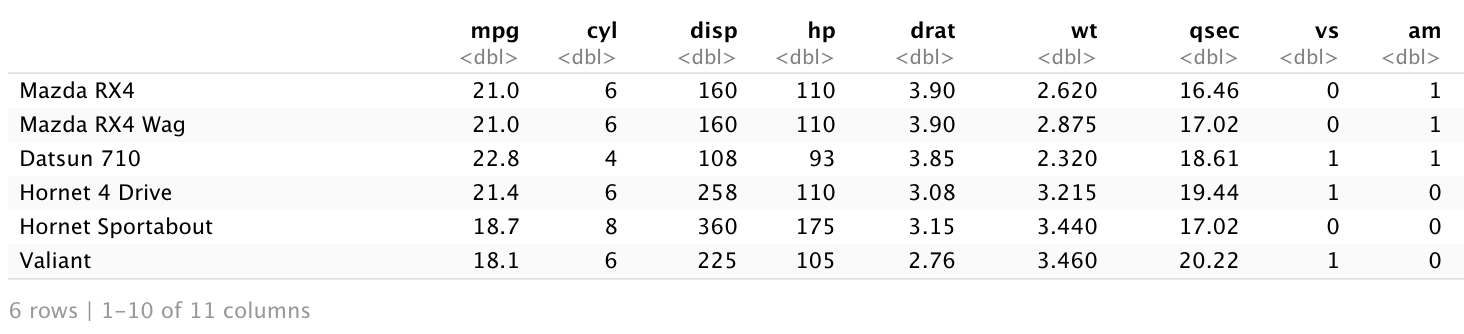It is also helpful to visualize the relationship between a vehicle’s weight and its fuel efficiency to confirm that the data meet the assumptions underlying your chosen statistical model. Below, we see that the relationship between vehicle weight and fuel efficiency is approximately linear, an assumption of linear regression models.

 a <- ggplot(data = mtcars, aes(x= wt, y = mpg)) +    geom_point() +    geom_smooth(method = lm) +    labs(x = "Weight",         y = "Miles per gallon",         title = "Relationship between weight and miles per gallon") show(a)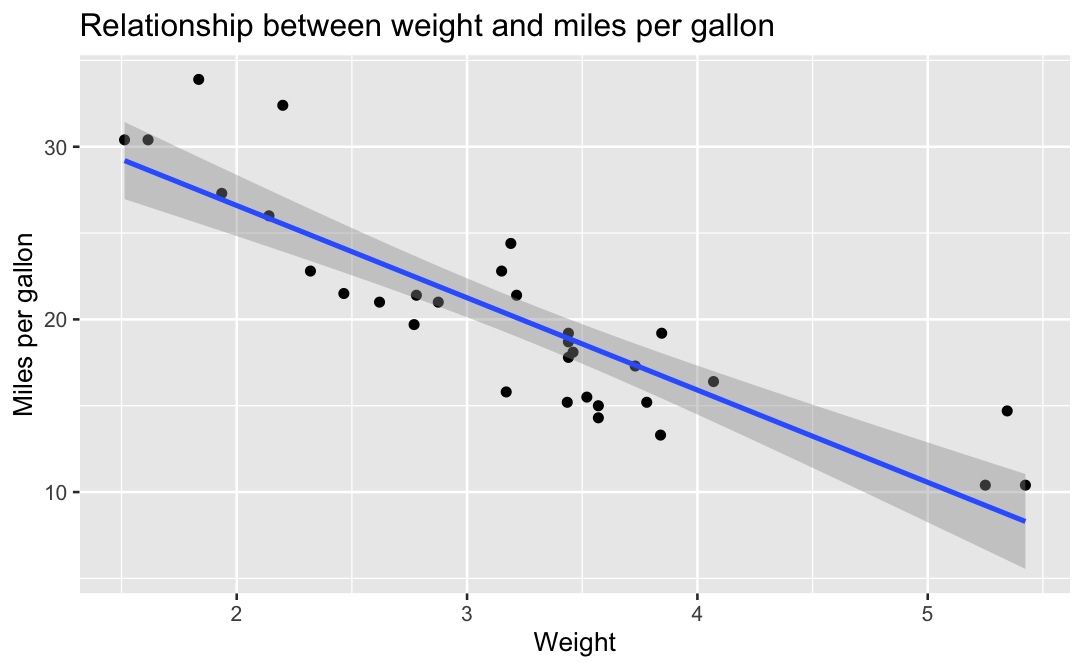Figure 1: A negative, linear relationship between Weight and Miles per Gallon

#### Step 2: Run regression models and review model output

We will graph four linear regression models. The first is an unadjusted model and the following three are adjusted for quarter-mile time (acceleration), horsepower, and quarter-mile time and transmission type, respectively.

 # Model 1: unadjusted m1 <- lm(mpg ~ wt, data = mtcars)  summary(m1) # Model 2: adjusted for quarter-mile time m2 <- lm(mpg ~ wt + qsec, data = mtcars)  summary(m2) # Model 3: adjusted for horsepower m3 <- lm(mpg ~ wt + hp, data = mtcars)  summary(m3) # Model 4: adjusted for quarter-mile time and transmission type m4 <- lm(mpg ~ wt + qsec + am, data = mtcars) summary(m4)

Below is the output from calling the summary() function on Model 4. As you can see, it contains a lot of information. Examining the output from each of the four models individually would be time-consuming for casual readers. Further, it would be tedious to compare results from one model to another. Let’s make these results easier to interpret with a forest plot.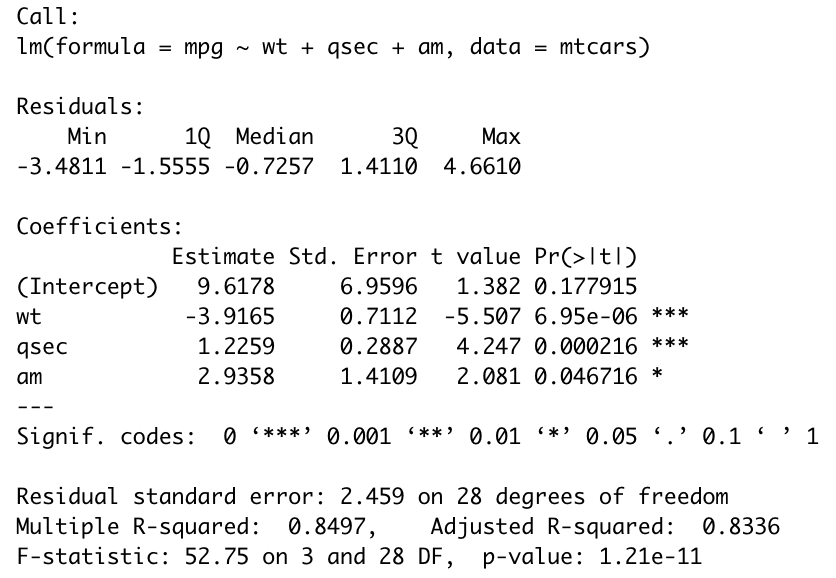#### Step 3: Summarize the regression output

The broom package can be used to summarize model output in a tibble, a type of data frame that is easy to use with ggplot2 and other R packages in the tidyverse . Since there is extra information in the summary() output shown above, this code chunk uses the tidy() function to extract only the estimate, standard error, test statistic, and p-value from each of the four regression models. The argument conf.int = TRUE adds the lower and upper confidence intervals (by default, 95%) to the tibble.

 df_m1 <- tidy(m1, conf.int = TRUE)  df_m2 <- tidy(m2, conf.int = TRUE)  df_m3 <- tidy(m3, conf.int = TRUE)  df_m4 <- tidy(m4, conf.int = TRUE)  head(df_m1)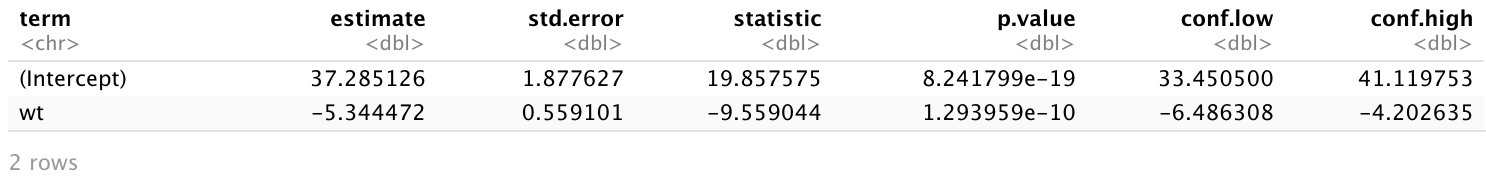The following code chunk extracts only the information needed for the forest plot and combines the results from the four models into one tibble with the model names.

 # Combine the tidy tibbles into one tibble and keep only the weight coefficient and 95% confidence interval from each model df <- rbind(df_m1, df_m2, df_m3, df_m4) %>%   filter(term == "wt") %>%   select(term,estimate,conf.low,conf.high) # Create a vector with labels for each model label <- c("Model 1", "Model 2", "Model 3", "Model 4") # Create a tibble with the model names, estimates, and 95% confidence intervals df <- cbind(label,df) head(df)

The following output from running head(), showing the estimate and 95% confidence interval for each model, is much easier to interpret than examining the full regression output of each model. The results show a negative relationship between a vehicle’s weight and its fuel efficiency.

In many papers, results of multiple models are compiled into a neat table. Even so, it may still be laborious to process all of the information at once. A visualization, like a forest plot, can make these results easier to understand.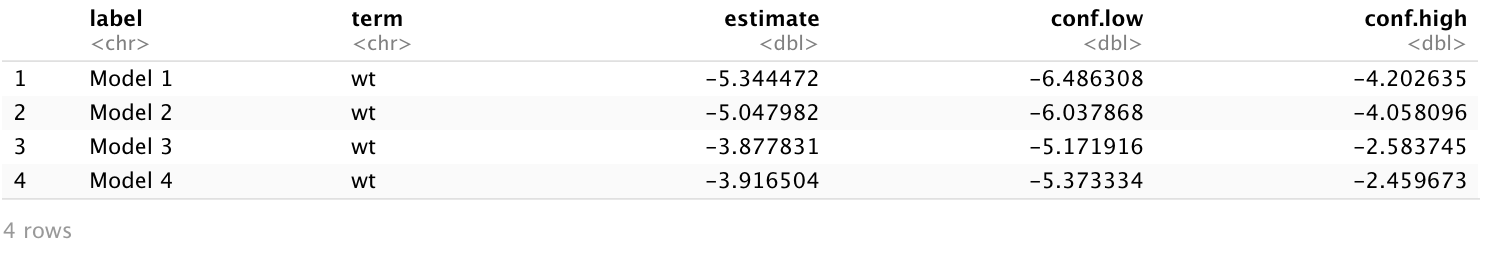Now that the results are neatly organized in a tibble, we will create two forest plots to convey the results. In Option 1, we use the ggplot2 package to create a forest plot. In Option 2, we use the forestplot package.

#### Step 4, Option 1: Create a forest plot using ggplot2

 df %>%   ggplot(aes(x=fct_rev(label),y=estimate,ymin=conf.low,ymax=conf.high)) +   geom_pointrange(color = "black", size = .5) +   geom_hline(yintercept = 0, color = "steelblue") +     coord_flip() +   xlab(" ") +   ylab("Coefficient (95% Confidence Interval)") +   labs(title ="Linear Regression Models Estimating the Effects of Vehicle Weight \n on Fuel Efficiency") +     theme(     plot.title = element_text(size = 14, face = "bold"),     axis.text.x = element_text(size = 12),     axis.text.y = element_text(size = 12))

The forest plot below depicts the regression results. We can quickly see that there is a negative relationship between a vehicle’s weight and its fuel efficiency and that the relationship is statistically significant in all four models. Additionally, we can easily compare the point estimates and confidence intervals.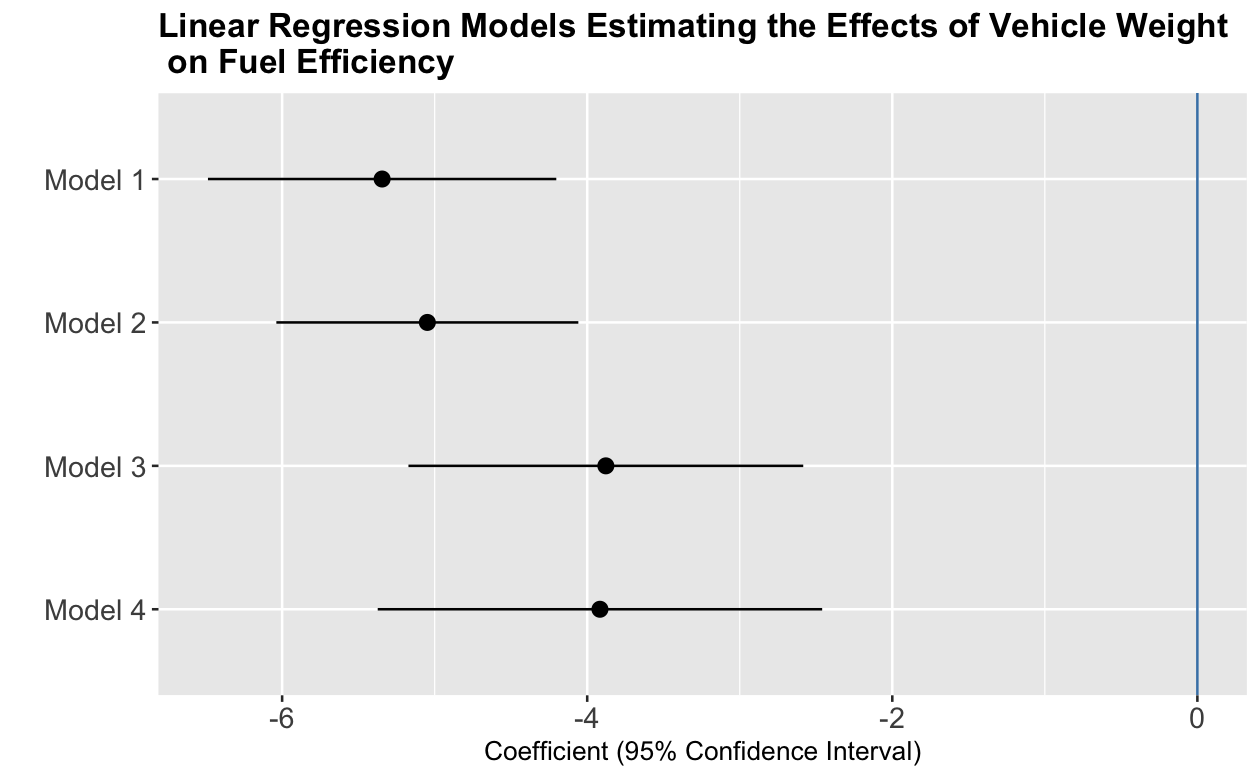Figure 2: Linear Regression Models Estimating the Effects of Vehicle Weight on Fuel Efficiency, created with ggplot2

#### Step 4, Option 2: Create a forest plot using forestplot

The forestplot package makes it easy to create forest plots. With just a few lines of code, it can graph the results of several regression models. Further, it can create highly customizable and complex forest plots (e.g., with multiple confidence intervals per row, custom confidence intervals, and more) .

 forestplot(labeltext = df\$label, mean = df\$estimate, lower = df\$conf.low, upper = df\$conf.high, xlab = "Adjusted Coefficients and 95% Confidence Intervals", boxsize = 0.1, col = fpColors(box = "black", line = "black", summary = "black", zero = "steelblue"), txt_gp = fpTxtGp(label  = gpar (cex = 1.0), xlab = gpar(cex = 1.0), ticks = gpar (cex = 1.0), title = gpar (cex = 1.0)), grid = TRUE, title = "Linear Regression Models Estimating the Effects of Vehicle Weight on \n Fuel Efficiency")

The forest plot below presents the same findings as above.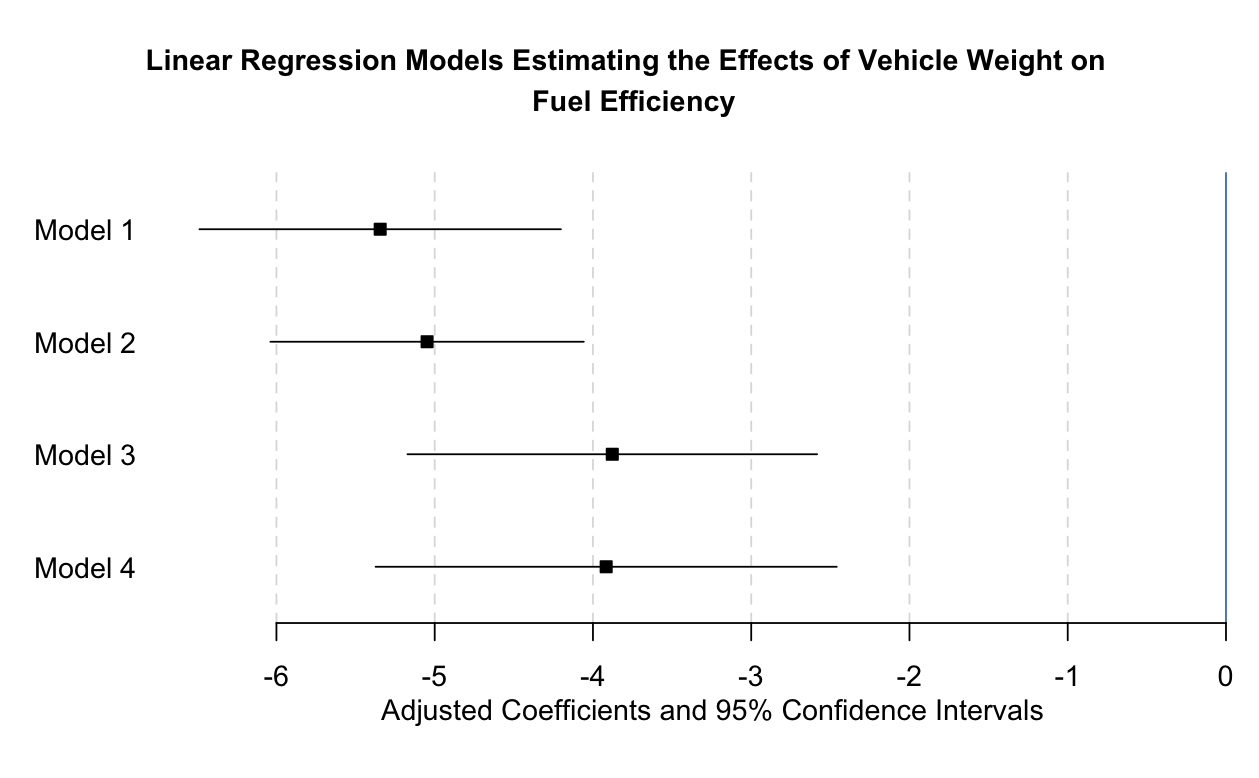Figure 3: Linear Regression Models Estimating the Effects of Vehicle Weight on Fuel Efficiency, created with forestplot

### Conclusion

In this tutorial, we created forest plots to visualize the results of linear regression models. These graphs can be used to show more complex results as well. For example, above we reported only the estimates and confidence intervals for a single independent variable (weight), but forest plots could be used to plot the estimates and confidence intervals of multiple variables from multiple models, too. With R, the possibilities for visualizing statistical estimates are endless!